# Summands

Find two summands of the number 42, so that its product is minimized.

Correct result:

a =  1
b =  41

#### Solution:

$b=42-a=42-1=41$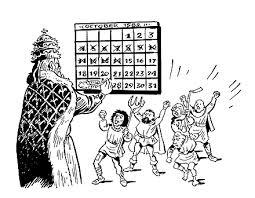We would be pleased if you find an error in the word problem, spelling mistakes, or inaccuracies and send it to us. Thank you!Tips to related online calculators
Do you have a linear equation or system of equations and looking for its solution? Or do you have quadratic equation?

## Next similar math problems:

• Prime divisorsFind 2/3 of the ratio of the sum and the product of all prime divisors of the number 120.
• Unknown numbersThe sum of two consecutive natural numbers and their triple is 92. Find these numbers.
• Odd numbersThe sum of four consecutive odd numbers is 1048. Find those numbers ...
• Divisibility by 12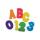Replace the letters A and B by digits so that the resulting number x is divisible by twelve /find all options/. x = 2A3B How many are the overall solutions?
• Four-digit numberFind also a four-digit number, which quadrupled written backwards is the same number.
• The King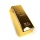The King wants to divide his sons equally. He has 42 rubies and 45 diamonds. How many sons and how will they share them?
• Z7-I-4 stars 4949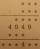Write instead of stars digits so the next write of product of the two numbers to be valid: ∗ ∗ ∗ · ∗ ∗ ∗ ∗ ∗ ∗ ∗ 4 9 4 9 ∗ ∗ ∗ ∗ ∗ ∗ 4 ∗ ∗
• Star equationWrite digits instead of stars so that the sum of the written digits is odd and is true equality: 42 · ∗8 = 2 ∗∗∗
• Unknown numberFind an unknown number of which 1/5 is 40 greater than one-tenth of that number.
• Sales of productsFor 80 pieces of two quality products a total sales is 175 Eur. If the first quality product was sold for n EUR per piece (n natural number) and the second quality product after 2 EUR per piece. How many pieces of the first quality were sold?
• 7 digit numberIf 3c54d10 is divisible by 330, what is the sum of c and d?
• Prime factorsFactor the number 6600 into the product of prime numbers.
• Two numbersThe sum of two numbers is 1. Identify this two numbers if you know that the half of first is equal to the third of second number.
• Four integersFnd four consecutive integers so that the product of the first two is 70 times smaller than the product of the next two.
• NumbersDetermine the number of all positive integers less than 4183444 if each is divisible by 29, 7, 17. What is its sum?
• Number ratio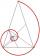Calculate two positive numbers that its ratio is 6:6 and difference was 0.
• Sports studentsThere are 120 athletes, 48 volleyball players, and 72 handball players at the school with extended sports training. Is it possible to divide sports students into groups so that the number in each group is the same and expressed by the largest possible num